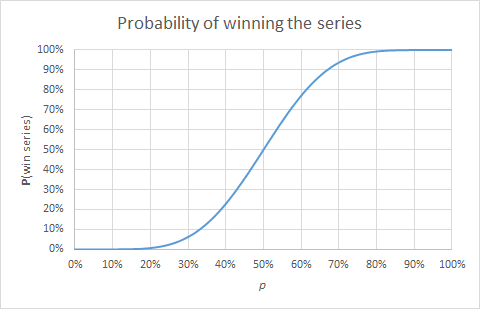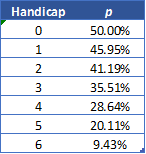## Handicapping a Race to 7

### If we give Karl a handicap of 2 games so that he only needs to win 5 games to win the series, whereas Richard needs to win 7, what is the single-game probability $p$ that gives each player a 50% chance of winning the series?

I've tagged this post with "Billiards" since the question came from the director of my pool league (who I hear is an avid GTM reader). Actually, what he really wants to know is the best way to set up the scoring, tie-breakers, and handicaps in one of the leagues, but in order to answer these questions, I wanted to start by looking at just one series and then extend that to the more general questions. This analysis really has nothing to do with pool though, so it would work for any other game as well.

#### Binomial Coefficients

To start out, we'll need the binomial coefficients $\binom{n}{k}$, which are read as "$n$ choose $k$." $\binom{n}{k}$ is the number of ways to choose $k$ items from a set of $n$ distinct items, for example the number of $k$-person boards of directors that can be chosen from $n$ candidates. Note that choosing the $k$ candidates who are included in the board is the same as choosing the $n-k$ who are not included, which means that $\binom{n}{k} = \binom{n}{n-k}$. The formula for the binomial coefficients is $$\dbinom{n}{k} = \dfrac{n!}{k! (n-k)!}$$ from which the above-mentioned symmetry is obvious. Note that even though this is a fraction, it always comes out to be an integer.

The binomial coefficients got their name from the fact that they are the coefficients in the expansion of binomials: $$(x+y)^n = \sum_{k=0}^{n}{\dbinom{n}{k} x^k y^{n-k}}$$ This is because in the product $(x+y)(x+y)...(x+y)$ (with $n$ factors of $(x+y)$), each factor of $(x+y)$ contributes either an $x$ or a $y$ to a factor in the sum. If you have $k$ $x$'s in a term, the other $n-k$ factors of $(x+y)$ must have contributed a $y$. There are $\binom{n}{k}$ ways to get $k$ $x$'s and $n-k$ $y$'s, hence the formula. If anyone wants more detail on that, just ask in the comments, and I'll give a more detailed explanation.

Most identities about binomial coefficients can be proved either by using the formula with the factorials, or via a combinatorial argument. For example, for integers $n$, $m$, and $k$ with $0 \leq k \leq m \leq n$, we have the following identity, known as the subset of a subset identity: $$\dbinom{n}{m} \dbinom{m}{k} = \dbinom{n}{k} \dbinom{n-k}{m-k}$$Algebraic proof: \begin{align} \dbinom{n}{m} \dbinom{m}{k} &= \dfrac{n!}{m! (n-m)!} \cdot \dfrac{m!}{k! (m-k)!} \\[3mm] &= \dfrac{n!}{k!(n-m)!(m-k)!} \\[3mm] &= \dfrac{n!}{k! (n-k)!} \cdot \dfrac{(n-k)!}{(n-m)! (m-k)!} \\[3mm] &= \dbinom{n}{k} \dbinom{n-k}{m-k} \tag*{\square} \end{align}Combinatorial proof:
The left side of the identity is the number of ways to choose a board of directors with $m$ members from $n$ candidates, and then choose $k$ executive members from the $m$. The right side counts the number of ways to choose $k$ executive members from the $n$ candidates and then choose the $m-k$ non-executive board members from the $n-k$ remaining candidates. These count the same thing, so the two sides must be equal. $\tag*{$\square$}$

#### Winning a series to 7

In order to win a series to 7, without needing to win by 2, Karl needs to win 7 games, with Richard winning anywhere from 0 to 6 games in the series. If Karl wins 7 games, and Richard wins 3 games (for example), there will be a total of 10 games in the series. The 3 games that Richard does win can come anywhere in the 10 games, except for the 10th game- if it did, then Karl would have already won 7 and the series would not have made it to 10 in the first place. So we can choose from the first $10-1=9$ games where Richard's 3 wins go.

The probability that Karl wins a given game is $p$, which means the probability that Richard beats Karl is $1-p$. Combining all this, we can see that the probability that Karl beats Richard in a race to 7, with Richard winning 3 games, is $$\binom{10-1}{3} p^7 (1-p)^3$$Since Karl can win the series with Richard winning anywhere from 0 to 6 games, the total probability that Karl wins the series is the sum over the possible outcomes, with the summation index $k$ being the number of wins Richard gets in the series: \begin{align} {\Bbb P}(\text{Karl wins the series}) &= \sum_{k=0}^{7-1}{\binom{7+k-1}{k} p^7 (1-p)^k} \\[3mm] &= \sum_{k=0}^{6}{\binom{6+k}{k} p^7 (1-p)^k} \end{align} Here's a graph of the probability that Karl wins the series, for different values of $p$:Not surprisingly, if there's a 50% chance that either player wins an individual game, then there's also a 50% chance that either player wins the series.

Now, let's say we give Karl a handicap of 2 games so that to win the series, Karl needs to win 5 games and Richard needs to win 7. More generally, if we call the handicap $H$, where $0 \leq H \leq 6$, then by the same reasoning as we used above, we get the modified formula: $${\Bbb P}(\text{Karl wins the series}) = \sum_{k=0}^{6}{\binom{6-H+k}{k} p^{7-H} (1-p)^k}$$ Now Karl only needs to win $7-H$ games, and so the total number of games in the series for a given value of $k$ wins for Richard, is $7-H+k$, with the $k$ losses once again being placed anywhere but the last game.

Here are the graphs of Karl's probabilities of winning the series given different values of $p$ and $H$ (you can click to expand the picture):

Now, I'd love to be able say we're done here, but the fact is that for some real Karl and Richard, we have no idea what the value of $p$ is unless we are lucky enough to have a history of, say, 100 games between these two players. And even then, they could have improved over time or gotten rusty or whatever so that games they played a few months ago aren't so telling now as to the value of $p$.

We do know that every player in the league is assigned a ranking (which directly determines the handicap against an opponent) which is certainly partly subjective and determined based on observation by a few very experienced players who run, and possibly play in, the league. Instead of trying to guess $p$ and then assigning the rankings, which would be useless in the absence of a large history of games between each set of two players, we can use the handicaps to back out the value of $p$ that makes the match 50-50. For example, if Karl and Richard's rankings are such that Karl gets a handicap of 3, we can see from the graph above that the match will be 50-50 if Karl's probability $p$ of winning an individual game is about 35.5%.

Using Excel's Goal Seek functionality, I've backed out the values of $p$ that make a 7-game series 50-50 for different handicaps:To test whether a player's handicap is appropriate, one could take all that player's games against opponents of different ranks and see what percentage of individual games he wins and how far off those percentages are from the table above (perhaps using a chi-square test for goodness of fit). If there are not enough games to do this analysis for individual players, then one could start by looking at the percentages for all games and then looking into the ranks furthest away from the table values and seeing if the stats of any particular player(s) are driving the difference. That's a bit of a manual exercise, but it's a start...

"Backing out" $p$ basically means finding the inverse of the function $f(p) = \sum_{k=0}^{6}{\binom{6-H+k}{k} p^{7-H} (1-p)^k}$. We know the function has an inverse because if you look at the graphs, they all pass the horizontal line test. To be more rigorous, they are polynomials in $p$ and thus continuous, and $f(0)=0$ and $f(1)=1$, so the intermediate value theorem tells us that $f$ is surjective. Furthermore, $f$ is increasing on the interval $p \in [0,1]$, so it's also one-to-one, and thus has an inverse.

Now, while Excel Goal Seek will certainly work for this, it would be kind of nice to know the inverse function, so I worked for a few hours today trying to figure out how to invert $f$, but couldn't quite figure it out. Maybe one of my more nerdy readers wants to take a crack at it? Otherwise, maybe I'll go post the question on stack exchange...

[Update 7/29/2015: there's been some confusion on the question I'm asking, so just to clarify, for the purposes of finding the inverse of $f(p)$, assume that the $H$ in the formula above is a constant. So technically, there is a different function $f$ for each value of $H$, which I guess you could call $f_{H}(p)$ or something.]

What I was trying (and maybe this isn't the best way to go about it) was to find a not-too-awful formula for the coefficient of $p^n$ in the sum above and then try to use the Lagrange inversion formula, but it gets a bit messy with all the binomial coefficients. I tried to expand the $(1-p)^k$, turning the sum into a double sum, then switch the order of summation (making sure to adjust the summation limits- the Iverson bracket is helpful at this step), and finally simplify somehow using identities of the binomial coefficients such as the subset of a subset identity above, but said simplification proved elusive, so I didn't even bother with the inversion formula.

Anyone have any thoughts on that or maybe a different way to find the inverse of $f(p)$? Let me know in the comments or email me, and I can provide more details of the computation I tried.

Thanks for reading, and I will try to do a follow-up on this post soon. As always, feel free to ask questions in the comments section.

1.